# Three-dimensional magneto-hydrodynamic flow over an exponentially stretching surface

Three-dimensional magneto-hydrodynamic flow over an exponentially stretching surface

Tarek G. EmamYasser Abd Elmaboud

Department of Mathematics, Faculty of Science and Arts, Khulais, University Of Jeddah, Saudi Arabia

Department of Mathematics, Faculty of Science, Ain-Shams University, Cairo, Egypt

Department of Mathematics, Faculty of Science, Al-Azhar University (Assiut Branch), Assiut, Egypt

Corresponding Author Email:
tarek.emam@sci.asu.edu.eg
Page:
987-996
|
DOI:
https://doi.org/ 10.18280/ijht.350435
|
Accepted:
|
Published:
31 December 2017
| Citation

OPEN ACCESS

Abstract:

The effect of magnetic field on the three-dimensional flow of a nanofluid induced by exponentially stretching surface is studied. The fluid temperature is also assumed to be distributed exponentially. The problem is solved using two methods: The first one is numerical and the second is homotopy analysis method (HAM). The obtained results are compared with some previous studies in some limiting cases and found to be in a good agreement. The results reveal the roles that the nano-particle volume fraction and magnetic field strength play in the variation of both of the fluid temperature and velocity.

Keywords:

heat transfer, MHD flow, stretching surface, three-dimensional flow

1. Introduction

Throughout Many industrial and technological applications involve boundary layer flow induced by the impulse motion of a moving extensible surface. Such applications include paper production, crystal growing, metal and polymer extrusion etc. Sakiadis  has introduced the problem of the boundary layer flow over a continuous solid surface that moves with a constant speed. Such problem has then been generalized by Crane for a stretching sheet. He has obtained a closed form exact solution for the velocity distribution. Grobka and Bobba  have studied the characteristics of the heat transfer of a continuous stretching surface with variable temperature. For the three-dimensional flow, Sajid et al  have investigated that problem over a stretching surface in a viscous fluid. While Lui and Anderson  have considered the heat transfer over a bidirectional stretching surface. Hayat et al  have discussed the chemically reactive flow of third grade fluid by an exponentially convected stretching sheet. Mushtaq et al  have introduced a numerical study for rotating flow of nanofluids caused by an exponentially stretching sheet. Nanofluid was first introduced by Choi  to describe the case of a fluid with suspended nanoparticles. Nanoparticles are nanometer sized particles or nanostructured materials engineered on the atomic scales. What is exciting about nanofluids is that they have an enhanced thermal conductivity and improved heat transfer properties. Even for low volume fractions (less than 0.1%) of nanoparticles, an enhancement up to 40% in thermal conductivity compared to base fluid has been found .The study of nanofluids is very important due to its wide range applications in the industrial processes. Nanofluids can be utilized to cool vehicle motors and welding equipment and to cool high warmth flux devices, for example, high-power microwave tubes and laser diode arrays. Because of the importance of nanofluids many researchers have studied nanofluids in several works (see -). To analyze the problem of nanofluids, it is assumed that the nanofluid is a single phase fluids, so a physical property of a nanofluid is considered as a function of the properties of both constitutes and concentrations. The study of the mechanism of the enhancement of heat transfer using nanofluids attracted many scientists. The contribution of them can be found in the book by Das et al . MHD covers the phenomena of electrically conducting fluids, where the velocity field and the magnetic field are coupled. MHD is employed in engineering to study the magnetic behavior of plasmas in fusion reactors, liquid-metal cooling of nuclear reactors and electromagnetic casting. The boundary layer flow under the application of a magnetic field has been studied by many researchers. Chamkha  has studied the three-dimensional natural convection flow caused by an inclined stretching surface in the presence of magnetic field. Mukhopadhyay et al  have investigated the problem of MHD boundary layer flow over a heated stretching sheet where the viscosity is considered to be variable. Zhang and Wang  have presented an exact self-similar solution for the problem of MHD boundary layer system for power-law fluids. Elbashbeshy et al  have considered the problem of unsteady laminar flow and heat transfer of an incompressible viscous fluid in the presence of thermal radiation, internal heat generation or absorbtion and magnetic field over an exponentially stretching surface subject to suction with an exponential temperature distribution. Recently, Khan et al  have studied the problem of three dimensional flow of a nanofluid induced by an exponentially stretching sheet. They have considered the effects of Brownian motion and thermophoretic diffusion of nano-particles in the considered mathematical model. Hayat et al  have discussed the simultaneous effects of convective conditions and nanoparticles on peristaltic motion. Hayat et al  have studied the influence of convective conditions in radiative peristaltic flow of pseudoplastic nanofluid in a tapered asymmetric channel. The effect of an inclined magnetic field on peristaltic flow of Williamson fluid in an inclined channel with convective conditions investigated by Hayat et al . Abd elmaboud  has studied the effect of varying magneto-hemodynamics flow in a semi-porous vertical channel with heat transfer. Boutra et al  have studied the problem of free convection enhancement within a nanofluid’ filled enclosure with square heaters. Rashad  has presented a study of unsteady nanofluid flow over an inclined stretching surface with convective boundary condition and anisotropic slip impact.

Much attention has not been given to the problem of the three-dimensional incompressible boundary layer flow of a nanofluid over a sheet that is stretched exponentially in two lateral directions with magnetic field even thought the study is very useful in many areas. The objective of this work is to study the effect of magnetic field on the three-dimensional flow of a nanofluid induced by exponentially stretching surface with heat transfer. In this study, the accuracy of the presented model is validated by comparing the obtained results by a numerical method and the HAM method (homotopy analysis method).

## 1.jpg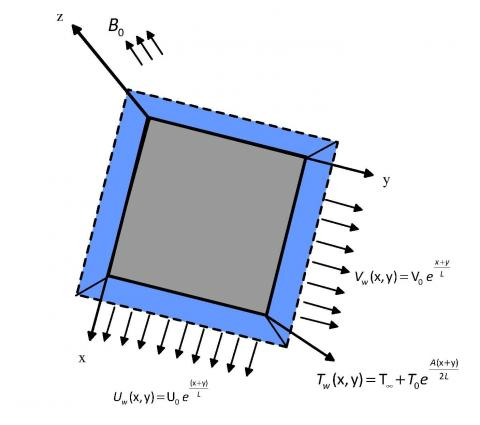Figure 1. Sketch of the physical problem

2. Formulation of the Problem

We study the case of a three dimensional incompressible boundary layer flow of a nanofluid over a sheet that is stretched exponentially in two lateral directions. A magnetic field of strength $B_{0}$ is applied normally to the sheet. We ignore the induced magnetic field comparing with the applied magnetic field as Reynolds number is considered to be small. The sheet is considered to be located at $z=0$ while the flow is confined to the region $z \geq 0$. The stretching velocities along $x-$ and $y-$ directions are $U_{w}(x, y)=U_{0} e^{\frac{x+y}{L}}$, and $V_{w}(x, y)=V_{0} e^{\frac{x+y}{L}}$ respectively. The temperature of the sheet is distributed as $T_{w}(x, y)=T_{\infty}+T_{0} e^{\frac{x+y}{2 L}}$, where $T_{\infty}$ is the ambient temperature, and $A$ is the temperature exponent parameter. The fluid velocity components are assumed to be $u, v$, and $\mathrm{w}$ along $x-, y-$ and $z-$directions respectively.

The governing equations are:

$\frac{\partial u}{\partial x}+\frac{\partial v}{\partial y}+\frac{\partial v}{\partial z}=0$         (1)

$u \frac{\partial u}{\partial x}+v \frac{\partial u}{\partial y}+w \frac{\partial u}{\partial z}=\left(\frac{\mu_{n f}}{\rho_{v f}}\right) \frac{\partial^{2} u}{\partial z^{2}}-\frac{\sigma_{n f} B_{0}^{2}}{\rho_{n f}} u$         (2)

$u \frac{\partial v}{\partial x}+v \frac{\partial v}{\partial y}+w \frac{\partial v}{\partial z}=\left(\frac{\mu_{n f}}{\rho_{n f}}\right) \frac{\partial^{2} v}{\partial z^{2}}-\frac{\sigma_{n f} B_{0}^{2}}{\rho_{n f}} v$         (3)

$u \frac{\partial T}{\partial x}+v \frac{\partial T}{\partial y}+w \frac{\partial T}{\partial z}=\alpha_{n f}\left(\frac{\partial^{2} T}{\partial z^{2}}\right)$        (4)

Subject to the boundary conditions:

$u=U_{w}(x, y), v=V_{w}(x, y), w=0, T=T_{w}(x, y)$ at $z=0$

$u=0, T \rightarrow T_{\infty}, \quad$ as $\quad z \rightarrow \infty$

where $\mu_{n f}$ the viscosity of nanofluid, $\rho_{n f}$ is the density of nanofluid, $\alpha_{n f}=\frac{K_{n f}}{\left(\rho C_{p}\right)_{n f}}$ is the nanofluid thermal diffusivity, $\sigma_{n f}$ is the electrical conductivity, and $B_{0}$ is the magnetic field. The nanofluid properties are related to the fluid properties as follows:

$\mu_{n f}=\frac{\mu_{f}}{(1-\phi)^{2.5}}, \cdot \rho_{n f}=(1-\phi) \rho_{f}+\phi \rho_{s}$

$\left(\rho C_{p}\right)_{n f}=(1-\phi)\left(\rho C_{p}\right)_{f}+\phi\left(\rho C_{p}\right)_{s}$

$\frac{K_{n f}}{K_{f}}=\frac{\left(K_{s}+2 K_{f}\right)-2 \phi\left(K_{f}-K_{s}\right)}{\left(K_{s}+2 K_{f}\right)+\phi\left(K_{f}-K_{s}\right)}$

$\frac{\sigma_{n f}}{\sigma_{f}}=1+\frac{3 \phi\left(\frac{\sigma_{s}}{\sigma_{f}}-1\right)}{\left(\frac{\sigma_{s}}{\sigma_{f}}+2\right)-\phi\left(\frac{\sigma_{s}}{\sigma_{f}}-1\right)}$

The index $n f$ stands for nanofluid, the index $f$ stands for the base fluid, and the index $s$ stands for solid.

Some thermo-physical properties of water and the nanoparticles $\cdot C u, \cdot A g$ and $A l_{2} O_{3}$ are listed in Table (1).

Table 1. Thermo-physical properties of nanofluids and base fluids.

 Property Water $C u$ $A g$ $A l_{2} O_{3}$ $C_{p}(J / k g K)$ 4179 385 235 765 $\rho\left(k g / m^{3}\right)$ 997.1 8933 10500 3970 $\kappa(W / m \cdot K)$ 0.613 400 429 40 $\alpha \times 10^{7}\left(m^{2} / s\right)$ 1.47 1163.1 1738.6 131.7 $\sigma(S / m)$ 5.5 $\times 10^{-6}$ 59.6 $\times 10^{-6}$ 63 $\times 10^{-6}$ 35 $\times 10^{-6}$

Using the dimensionless variables:

$u=U_{0} e^{\frac{x+y}{L}} f^{\prime}, v=U_{0} e^{\frac{x+y}{L}} g^{\prime}$

$w=-\sqrt{\frac{v_{f} U_{0}}{2 L}} e^{\frac{x+y}{2 L}}\left(f+\eta f^{\prime}+g+\eta g^{\prime}\right)$

$T=T_{\infty}+T_{0} e^{\frac{A(x+y)}{2 L}} \theta, \eta=\sqrt{\frac{U_{0}}{2 v_{f} L} e^{\frac{x+y}{2 L}} z}$             (5)

Equation (1) is identically satisfied, while equations (2)-(4) are transformed to the form:

$f^{\prime \prime \prime}+\frac{\Gamma_{1}}{H}\left[-2 f^{\prime}\left(f^{\prime}+g^{\prime}\right)+f^{\prime \prime}(f+g)\right]-\frac{M \Gamma_{4}}{B H} f^{\prime}=0$           (6)

$g^{\prime \prime \prime}+\frac{\Gamma_{1}}{H}\left[-2 g^{\prime}\left(f^{\prime}+g^{\prime}\right)+g^{\prime \prime}(f+g)\right]-\frac{M \Gamma_{4}}{B H} g^{\prime}=0$           (7)

$\theta^{\prime \prime}-A P r \frac{\Gamma_{2}}{\Gamma_{3}}\left(f^{\prime} \theta+g^{\prime} \theta\right)+\operatorname{Pr} \frac{\Gamma_{2}}{\Gamma_{3}}\left(f \theta^{\prime}+g \theta^{\prime}\right)=0$           (8)

while the boundary conditions take the form:

$f(0)=g(0)=0, f^{\prime}(0)=1, g^{\prime}(0)=\lambda, \theta(0)=1$

$f^{\prime} \rightarrow 0, g^{\prime} \rightarrow 0, \theta \rightarrow 0,$ as $\eta \rightarrow \infty$         (9)

where,

$\Gamma_{1}=1-\phi+\phi \frac{\rho_{s}}{\rho_{f}}$,

$H=\frac{1}{(1-\phi)^{2.5}}$,

$B=e^{\frac{x+y}{L}}=e^{X+Y}, \Gamma_{2}=1-\phi+\phi \frac{\left(\rho C_{p}\right)_{s}}{\left(\rho C_{p}\right)_{f}}$

$\Gamma_{3}=\frac{K_{n f}}{K_{f}}=\frac{K_{s}+2 K_{f}-2 \phi\left(K_{f}-K_{s}\right)}{K_{s}+2 K_{f}+\phi\left(K_{f}-K_{s}\right)}$

$\Gamma_{4}=1+\frac{3 \phi\left(\frac{\sigma_{s}}{\sigma_{f}}-1\right)}{\left(\frac{\sigma_{s}}{\sigma_{f}}+2\right)-\phi\left(\frac{\sigma_{s}}{\sigma_{f}}-1\right)}$

$M=\frac{2 L B_{0}^{2} \sigma_{f}}{U_{0} \rho_{f}}$ is the magnetic number,  $P r=\frac{v_{f}}{\alpha_{f}}$ is the Prandtle number, and $\lambda=\frac{V_{0}}{U_{0}}$ is the velocity ratio.

3. Solutions and Results

The mathematical model is solved by two methods: The first one is numerical and the second is homotopy analysis method (HAM). Comparisons between the results obtained from the two methods are given for some values of parameters in tables (3-6).

3.1 Numerical solutions

Equations (6)-(8) are transformed into the following simultaneous system of first order differential equations:

$y_{1}^{\prime}=y_{2}$            (10)

$y_{2}^{\prime}=y_{3}$            (11)

## 2.png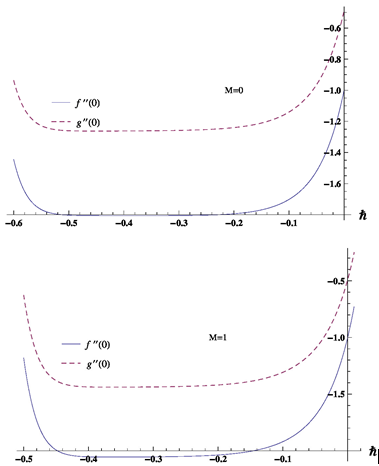Figure 2. $\hbar$-curve for the function $f$ and $g$ at 14th order approximation for different values of the magnetic parameter $M$ for $C u$-water fluid at $\phi=0.4, X=0.1, Y=0.1, \lambda=0.7, P r=6.2, A=0.8$.

$y_{3}^{\prime}=\frac{\Gamma_{1}}{H}\left[2 y_{2}\left(y_{2}+y_{5}\right)-y_{3}\left(y_{1}+y_{4}\right)\right]+\frac{\Gamma_{4} M}{B H} y_{2}$            (12)

$y_{4}^{\prime}=y_{5}$              (13)

$y_{5}^{\prime}=y_{6}$              (14)

$y_{6}^{\prime}=\frac{\Gamma_{1}}{H}\left[2 y_{5}\left(y_{2}+y_{5}\right)-y_{6}\left(y_{1}+y_{4}\right)\right]+\frac{\Gamma_{4} M}{B H} y_{5}$           (15)

$y_{7}^{\prime}=y_{8}$              (16)

$y_{8}^{\prime}=A \operatorname{Pr} \frac{\Gamma_{2}}{\Gamma_{3}}\left(y_{2} y_{7}+y_{5} y_{7}\right)-\operatorname{Pr} \frac{\Gamma_{2}}{\Gamma_{3}}\left(y_{1} y_{8}+y_{4} y_{8}\right)$               (17)

subject to the initial conditions

$y_{1}(0)=0, y_{2}(0)=1, y_{4}(0)=0, y_{5}(0)=\lambda, y_{7}(0)=1$

$y_{3}(0)=s_{1}, y_{6}(0)=s_{2}, y_{8}(0)=s_{3}$          (18)

## 3.png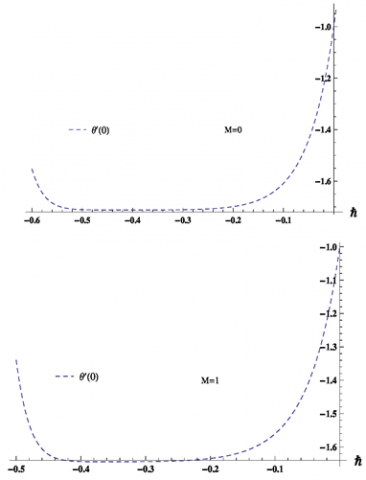where $y_{1}=f(\eta), y_{4}=g(\eta),$ and $y_{7}=\theta(\eta)$.

Figure 3. $\hbar$-curve for the function $\theta$ at 14th order approximation for different values of the magnetic parameter $\mathbf{M}$ for $C u$-water fluid at $\phi=0.4, X=0.1, Y=0.1, \lambda=0.7, P r=6.2, A=0.8$.

The constants $S_{1}, S_{2}$ and $S_{3}$ are priori unknowns that will be determined as a part of the solution. Using Mathematica a function $F\left[s_{1}, s_{2}, s_{3}\right]=$ NDSolve[System(10) $-(17) ]$ is defined. Given that $y_{2}(\eta), y_{5}(\eta)$ and $y_{7}(\eta)$ should tend to zero as $\eta$ tends to $\mathrm{O}$, the values of $s_{1}, s_{2}$ and $s_{3}$ are determined upon solving the equations $y_{2}\left(\eta_{\max }\right)=0, y_{5}\left(\eta_{\max }\right)=0$, and $y_{7}\left(\eta_{\max }\right)=0$, where a suitable value of $\eta$ is taken and then increased to reach $\eta_{\max }$ where the difference between two successive values of any of $s_{1}, s_{2}$ and $s_{3}$ is less then or equal to $10^{-6}$. Upon obtaining the values of $S_{1}, S_{2}$ and $\cdot s_{3}$ the closed system $(10)-(17)$ is solved numerically using the Mathematica function NDSolve . To validate this method, a comparison is made between the results obtained using this method and the results obtained in previous studies(Table (2)) in some special cases. From the table one can find that the present results are in a good agreement with previous ones.

Table 2. Comparison between numerical results obtained and previous published results in the case of 2D($g=0$) at$\phi=X=Y=M=0,$ and $\cdot A=1$

 Present Results Ref  Abs. Error Ref  Abs. Error Ref  Abs. Error 0.72 0.76728 0.76728 0.00000 1 0.95478 0.95478 0.00000 0.95480 0.00002 0.95478 0.00000 2 147146 1.47150 0.00004 1.47145 0.00001 3 1.86907 1.86910 0.00003 1.86910 0.00003 1.86905 0.00002 5 2.50013 2.50010 0.00003 2.50012 0.00002 10 3.66037 3.66040 0.00003 3.66036 0.00001

## 4.png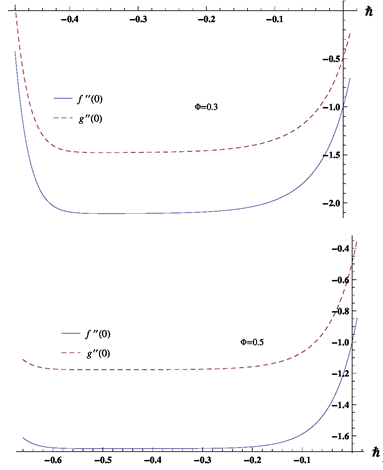Figure 4. $\hbar$-curve for the function $f$ and 8 at 20th order approximation for different values of $\phi$  for $C u$-water fluid at $M=0.5, X=0.1, Y=0.1, \lambda=0.7, P r=6.2, A=0.4$.

3.2 The ham solution of the problem

It’s well known that the HAM depends on initial solutions for different variables. Moreover, such solutions should satisfy the boundary conditions. We choose the initial approximations of $f, g,$ and $\cdot \theta$ as follow:

$f_{0}=1-\exp (-\eta)$         (19)

$g_{0}=1-\exp (-\lambda \eta)$         (20)

$\theta_{0}=\exp (-\eta)$           (21)

and the linear auxiliary operators are

$L_{1}(f)=\frac{d^{3} f}{d \eta^{3}}-\frac{d f}{d \eta}, L_{2}(g)=\frac{d^{3} g}{d \eta^{3}}-\frac{d g}{d \eta}$ and $\cdot L_{3}(\theta)=\frac{d^{2} \theta}{d y^{2}}-\theta$

where the auxiliary operators satisfy the equations:

$L_{1}\left(c_{1} \exp (\eta)+c_{2} \exp (-\eta)+c_{3}\right)=0$            (22)

$L_{2}\left(c_{4} \exp (\eta)+c_{5} \exp (-\eta)+c_{6}\right)=0$           (23)

$L_{2}\left(c_{7} \exp (\eta)+c_{8} \exp (-\eta)\right)=0$              (24)

where $c_{1}, c_{2}, c_{3}, c_{4}, c_{5}, c_{6}, c_{7} \quad, \quad$ and $\cdot c_{8}$ are constants. The equations of deformation for the zeroth-order with a non-zero auxiliary parameter $\hbar$, can be set as follow:

$(1-p)\left[L_{1}(f(\eta ; p))-L_{1}\left(f_{0}(\eta)\right)\right]=p \hbar N_{1}(f(\eta ; p))$           (25)

$(1-p)\left[L_{2}(g(\eta ; p))-L_{2}\left(g_{0}(\eta)\right)\right]=p \hbar N_{2}(g(\eta ; p))$          (26)

$(1-p)\left[L_{3}(\theta(\eta ; p))-L_{3}\left(\theta_{0}(\eta)\right)\right]=p \hbar N_{3}(\theta(\eta ; p))$           (27)

## 5.png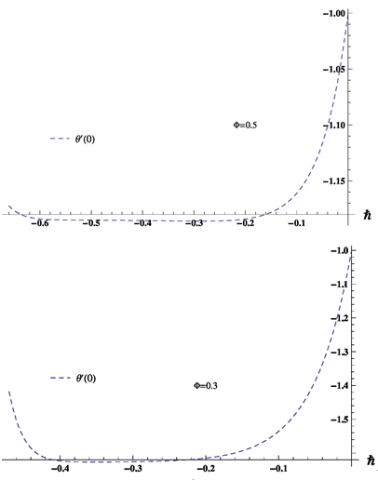Figure 5. $\hbar$-curve for the function $\theta$ at 20th order approximation for different values of $\phi$ for $^{C} u$-water fluid at $M=0.5, X=0.1, Y=0.1, \lambda=0.7, \mathrm{Pr}=6.2, A=0.4$.

The boundary conditions take the form

$f(\eta ; p)=g(\eta ; p)=0, \quad f^{\prime}(\eta ; p)=1, \quad g^{\prime}(\eta ; p)=\lambda, \theta(\eta ; p)=1,$ at $\eta=0$

$f^{\prime}(\eta ; p) \rightarrow 0, \quad g^{\prime}(\eta ; p) \rightarrow 0, \quad \theta(\eta ; p) \rightarrow 0, \quad$ as $\quad y \rightarrow \infty$         (28)

where $N_{1}(f(\eta ; p)), \cdot N_{2}(g(\eta ; p))$ and $\cdot N_{3}(\theta(\eta ; p))$ are defined as:

$N_{1}(f(\eta ; p))=f^{\prime \prime \prime}(\eta ; p)+\frac{\Gamma_{1}}{H}\left[f^{\prime \prime}(\eta ; p)(f(\eta ; p)+g(\eta ; p))\right.$

$-2 f^{\prime}(\eta ; p)\left(f^{\prime}(\eta ; p)+g^{\prime}(\eta ; p)\right) ]-\frac{M \Gamma_{4}}{BH} f^{\prime}(\eta ; p)$          (29)

$N_{2}(f(\eta ; p))=g^{\prime \prime \prime}(\eta ; p)+\frac{\Gamma_{1}}{H}\left[g^{\prime \prime}(\eta ; p)(f(\eta ; p)+g(\eta ; p))\right.$

$-2 g^{\prime}(\eta ; p)\left(f^{\prime}(\eta ; p)+g^{\prime}(\eta ; p)\right) ]-\frac{M \Gamma_{4}}{B H} g^{\prime}(\eta ; p)$          (30)

$N_{3}(\theta(\eta ; p))=$

$\theta^{\prime \prime}(\eta ; p)-\frac{A P r \Gamma_{2}}{\Gamma_{2}}\left[f^{\prime}(\eta ; p) \theta(\eta ; p)+g^{\prime}(\eta ; p) \theta(\eta ; p)\right]$$+\frac{\operatorname{Pr} \Gamma_{2}}{\Gamma_{3}}\left[f(\eta ; p) \theta^{\prime}(\eta ; p)+g(\eta ; p) \theta^{\prime}(\eta ; p)\right]$         (31)

As $p$, varies from 0 to 1, the functions $f(\eta ; p), \cdot g(\eta ; p)$ and $\theta(\eta ; p)$ vary from $f_{0}(\eta), \cdot g_{0}(\eta)$ and $\cdot \theta_{0}(\eta)$ to $f(\eta)$, $g(\eta)$ and $\theta(\eta)$ respectively. By using Taylor’s theorem $f(\eta ; p), g(\eta ; p)$ and $\cdot \theta(\eta ; p)$ can be expanded in a power series of $p$ as follows:

$f(\eta ; p)=f_{0}(\eta)+\sum_{m=1}^{\infty} p^{m} f_{m}(\eta)$               (32)

$g(\eta ; p)=g_{0}(\eta)+\sum_{m=1}^{\infty} p^{m} g_{m}(\eta)$           (33)

$\theta(\eta ; p)=\theta_{0}(\eta)+\sum_{m=1}^{\infty} p^{m} \theta_{m}(\eta)$          (34)

where

$f_{m}(\eta)=\frac{1}{m !} \frac{\partial^{m} f(\eta ; p)}{\partial p^{m}}, g_{m}(\eta)=\frac{1}{m !} \frac{\partial^{m} g(\eta ; p)}{\partial p^{m}}$  and

$\theta_{m}(\eta)=\frac{1}{m !} \frac{\partial^{m} \theta(\eta ; p)}{\partial p^{m}}$              (35)

The selection of the parameter $\hbar$ is taken in such a way that the series (32 - 34) are convergent at $p=1$ so we have:

$f(\eta)=f_{0}(\eta)+\sum_{m=1}^{\infty} f_{m}(\eta)$            (36)

$g(\eta)=g_{0}(\eta)+\sum_{m=1}^{\infty} g_{m}(\eta)$             (37)

$\theta(\eta)=\theta_{0}(\eta)+\sum_{m=1}^{\infty} \theta_{m}(\eta)$          (38)

Differentiating Eqs. (25)-(27) $m$ times with respect to $p$ and then dividing them by $m !$ and finally setting $p=0$, we have the following $m$th-order deformation problem:

$L_{1}\left[f_{m}(\eta)-\chi_{m} f_{m-1}(\eta)\right]=\hbar N_{1}^{m}(f(\eta ; p))$         (39)

$L_{2}\left[g_{m}(\eta)-\chi_{m} g_{m-1}(\eta)\right]=\hbar N_{2}^{m}(g(\eta ; p))$           (40)

$L_{2}\left[\theta_{m}(\eta)-\chi_{m} \theta_{m-1}(\eta)\right]=\hbar N_{3}^{m} \theta(\eta ; p)$            (41)

where,

$N_{1}^{m}(f)=f_{m-1}^{\prime \prime \prime}+\frac{\Gamma_{1}}{H}\left[\sum_{i=0}^{m-1} f_{i}^{\prime \prime} f_{m-i-1}+\sum_{i=0}^{m-1} f_{i}^{\prime} g_{m-i-1}\right.$

$-2\left[\sum_{i=0}^{m-1} f_{i}^{\prime} f_{m-i-1}^{\prime}+\sum_{i=0}^{m-1} f_{i}^{\prime} g_{m-i-1}^{\prime}\right] ]-\frac{M \Gamma_{4}}{B H} f_{m-1}^{\prime}$               (42)

\begin{aligned} N_{2}^{m}(g) &=g_{m-1}^{\prime \prime \prime}+\frac{1}{H}\left[\sum_{i=0}^{m-1} g_{i}^{\prime \prime} f_{m-i-1}+\sum_{i=0}^{m-1} g_{i}^{\prime \prime} g_{m-i-1}\right.\\ &-2\left[\sum_{i=0}^{m-1} g_{i}^{\prime} f_{m-i-1}^{\prime}+\sum_{i=0}^{m-1} g_{i}^{\prime} g_{m-i-1}^{\prime}\right] ]-\frac{M \Gamma_{4}}{B H} g_{m-1}^{\prime} \end{aligned}            (43)

\begin{aligned} N_{3}^{m}(\theta)=& \theta_{m-1}^{\prime \prime}-\frac{A P r \Gamma_{2}}{\Gamma_{3}}\left[\sum_{i=0}^{m-1} f_{i}^{\prime} \theta_{m-i-1}+\sum_{i=0}^{m-1} g_{i}^{\prime} \theta_{m-i-1}\right] \\ &+\frac{\operatorname{Pr} \Gamma_{2}}{\Gamma_{3}}\left[\sum_{i=0}^{m-1} f_{i} \theta_{m-i-1}^{\prime}+\sum_{i=0}^{m-1} g_{i} \theta_{m-i-1}^{\prime}\right] \end{aligned}          (44)

where

$\chi_{m}=\left\{\begin{array}{l}{0, m \leq 1} \\ {1, m>1}\end{array}\right.$              (45)

with the boundary conditions

$f_{m}=g_{m}=0, \quad f_{m}^{\prime}=0, \quad g_{m}^{\prime}=0, \quad \theta_{m}=0,1.5 \quad$ at $\quad \eta=0$

$f_{m}^{\prime} \rightarrow 0, \quad g_{m}^{\prime} \rightarrow 0, \quad \theta_{m} \rightarrow 0,3.7 \quad$ as $\eta \rightarrow \infty$         (46)

With the aid of MATHEMATICA software we obtain the solution of these equations.

## 6.jpg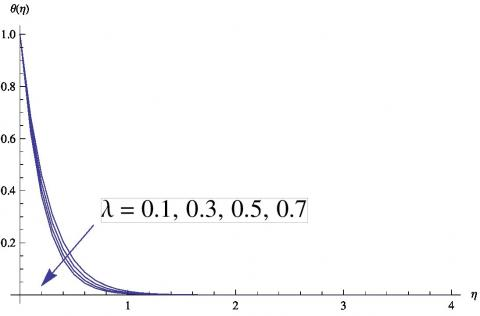Figure 6. Variation of temperature profiles with $\lambda,$ where $X=1, Y=1, \phi=0.01, A=2, M=0.01,$ and $\cdot \operatorname{Pr}=6.2$

## 7.jpgFigure 7. Variation of the velocity $x-$ components with $\lambda$, where $X=1, Y=1, \cdot \phi=0.01, \cdot A=2, \cdot M=0.01$ and $\cdot P r=6.2$

4. Convergence Region

Figures (2)-(5) show the values that the auxiliary parameter $\hbar$ take in order to get convergent solutions for the fluid velocities and temperature. From such figures one can conclude the following:

• For different values of the magnetic number $M$, convergent solutions for the velocities are obtained if $-0.4 \leq \hbar \leq-0.28$. While all other parameters are fixed.

• Convergent solution for temperature is obtained when $-0.4 \leq \hbar \leq-0.22$ for different values of the magnetic number $M$. While all other parameters are fixed.

• For the volume fraction, convergent solutions for the velocities and temperature are obtained if  and  respectively. While all other parameters are fixed.

The results obtained by this method is compared with the numerical results for some values of parameters and found to be in a good agreements as shown in tables (3-6).

## 8.jpgFigure 8. Variation of the velocity $y-$ components with $\lambda$, where $X=1, Y=1, \phi=0.01, A=2, M=0.01,$ and $P r=6.2$

## 9.jpg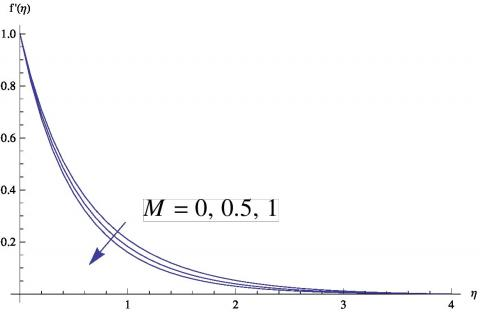Figure 9. Variation of the velocity $x-$ components with $M$, where $X=0.1, Y=0.1, \phi=0.03, A=2, \lambda=0.7,$ and $P r=6.2$

Table 3. Comparison between HAM and numerical values for $C u-water$  at $\operatorname{Pr}=6.2, M=0.01, \phi=0.4, A=0.2, X=0.1, Y=0.1$

 HAM NUM Abs. Error 0.9 -1.9084 -1.7172 -1.3288 -1.9251 -1.7326 -1.3123 0.0167 0.0154 0.0165 0.7 -1.8062 -1.2628 -1.2572 -1.8212 -1.2748 -1.2412 0.0150 0.0120 0.0160 0.3 -1.5824 -0.4701 -1.1004 -1.5933 -0.4780 -1.0851 0.0109 0.0079 0.0153

Table 4. Comparison between HAM and numerical values for $C u-water$ at $\operatorname{Pr}=6.2, M=0.01, \phi=0.4, \lambda=0.7, X=0.1, Y=0.1$

 HAM NUM Abs. Error A 0.3 -1.8062 -1.2628 -1.3383 -1.8052 -1.2636 -1.3383 0.0010 0.0008 0.0000 0.5 -1.8062 -1.2628 -1.4935 -1.8052 -1.2636 -1.4941 0.0010 0.0008 0.0006 0.8 -1.8062 -1.2628 -1.7109 -1.8052 -1.2636 -1.7112 0.0010 0.0008 0.0003

Table 5. Comparison between HAM and numerical values for $C u-water$ at $P r=6.2, A=0.3, \phi=0.4, \lambda=0.7, X=0.1, Y=0.1$

 HAM NUM Abs. Error M 0 -1.8043 -1.2614 -1.3388 -1.8051 -1.2636 -1.3387 0.0008 0.0022 0.0001 0.5 -1.8973 -1.3272 -1.3154 -1.8994 -1.3296 -1.3155 0.0021 0.0024 0.0001 1 -1.9856 -1.3891 -1.2936 -1.3921 -1.2937 -1.7112 0.0031 0.0030 0.0001

Table 6. Comparison between HAM and numerical values for $C u-water$ at $\operatorname{Pr}=6.2, A=0.3, M=0.1, \lambda=0.9, X=0.1, Y=0.1$

 HAM NUM Abs. Error 0.2 -2.1980 -1.9796 -1.9645 -2.1715 -1.9543 -1.9603 0.0265 0.0253 0.0042 0.3 -2.1120 -1.9012 -1.6577 2.1000 -1.8909 -1.6559 0.0120 0.0103 0.0018 0.4 -1.9287 -1.7359 -1.4111 -1.9266 -1.7340 -1.4108 0.0021 0.0019 0.0003
5. Discussions

Here we consider one type of nanofluids that is Cu-water. The effect of the velocity ratio on the fluid temperature is exhibited in figure (6). It can be observed that the fluid temperature decreases with the increase of. The variation of the velocity x- component with is shown in figure (7), while figure (8) shows the variation of the velocity y-component with. One can see that the increase of results in an increase in but a decrease in. This behavior can be understood in view of the effect of on the stretching of the surface. That is the augmentation of indicates a larger stretching rate in y-direction which results in increasing and deceasing.

## 10.jpg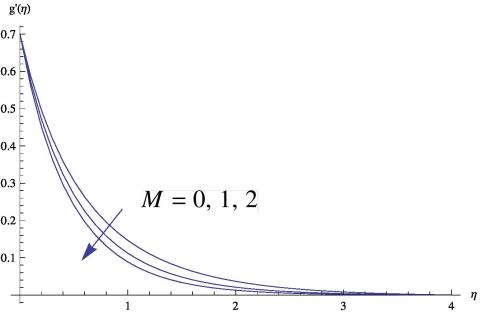Figure 10. Variation of the velocity $y-$ components with M, where $x=0.1, Y=0.1, \phi=0.03, A=2, \lambda=0.7,$ and $P_{r}=6.2$

## 11.jpg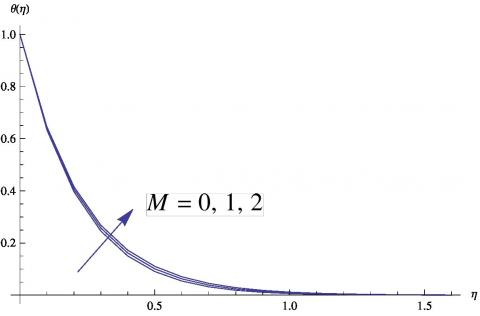Figure 11. Variation of temperature profiles with $M$, where $X=0.1, Y=0.1,-\phi=0.03, \cdot A=2, \cdot \lambda=0.7$ and $\cdot P r=6.2$

## 12.png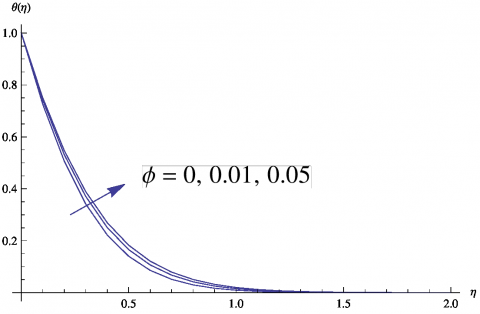Figure 12. Variation of temperature profiles with $\phi$, where $X=1, Y=1,-M=0.01, \cdot A=0.5,-\lambda=0.7$ and $\cdot P r=6.2$

Figures (9) and (10) exhibit the effect of the magnetic parameter of and respectively. It can be noted that the fluid velocity decreases with the increase of. The increase of elevates the Lorentz force in the boundary layer. In fact Lorentz force opposes the flow and this results in reducing the fluid motion. As a result of reducing the fluid velocity due to increasing, the fluid temperature of the boundary layer increases with the increase of as shown in Figure (11).

## 13.pngFigure 13. Variation of the velocity $x-$ components with$\phi$, where $X=1, Y=1,-M=0.01, \cdot A=0.5,-\lambda=0.7$ and $P r=6.2$

## 14.jpg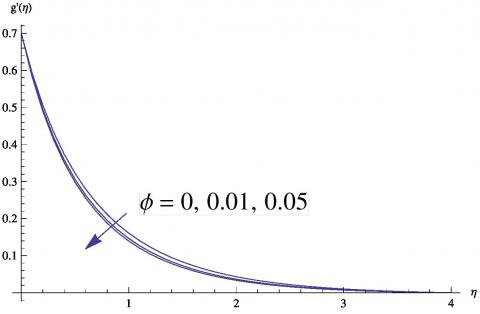Figure 14. Variation of the velocity $y-$ components with $\phi$, where $X=1, Y=1, \cdot M=0.01, \quad A=0.5, \cdot \lambda=0.7$ and $\cdot P r=6.2$

## 15.jpgFigure 15. Variation of temperature profiles with $A$, where $X=1, Y=1, \cdot M=0.01, \phi=0.02, \quad \lambda=0.7$ and $\cdot P r=6.2$

## 16.jpg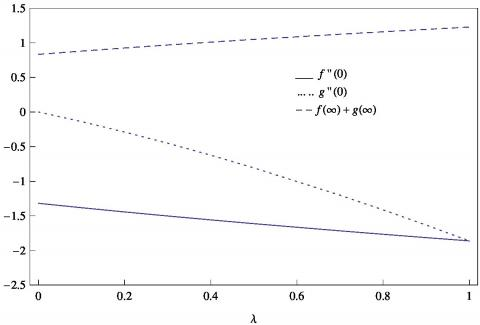Figure 16. Variations in wall shear stresses and entrainment velocity with

From the results shown in table, one can see that the wall heat flux increases with the increase of the nano-particle volume fraction. From figure (12) we find that the fluid temperature increases with the increase of and however it is greater than that of the corresponding case of pure fluid (). Such results can be justified as follows: The immersing of nano-particles into pure fluid improves the thermal conductivity of the fluid and the enhancement of increases the frictional forces inside the fluid and that between the fluid and the surface. The nano-particles decelerate the fluid motion and consequently the values of and decrease with the increase of as shown in figures (13) and (14) respectively.

The expansion coefficient has a considerable effect on the fluid temperature as shown in Figure (15). The fluid temperature decreases with the increase of the value of. In Figure (16) we can see that the sheer stress in both of and directions decrease with the increase of. Consequently the entrainment velocity increases with the increase of.

6. Concluding Remarks

The problem of three-dimensional MHD flow over an exponentially stretching surface is studied using a numerical method as well as a HAM method. The followings are concluded:

• The obtained results for some parameters show that the two methods used in this article are in a good agreement.

• The fluid temperature decreases with the increase of the velocity ratio as well as the expansion coefficient.

• The fluid temperature increases with the increase of the nanofluid volume fraction as well as the magnetic parameter.

• Both of the fluid velocity components decreases with the increase of the magnetic parameter as well as the nanoparticles fractional volume.

• The increase of the velocity ratio increases the component of the velocity but decreases the component of the velocity.

Acknowledgment

The authors wish to express their gratitude to the reviewers for the useful comments which helped to improve the present article.

Nomenclature
 $x, y, z$ Cartesian coordinate $U_{w}, V_{w}$ the stretching velocities $T_{w}$ the temperature of the sheet $T_{\infty}$ the ambient temperature $u, v, w$ The fluid velocity component along $x, y, z$ respectively $A$ the temperature exponent parameter $\mu_{n f}$ the viscosity of nanofluid $\rho_{n f}$ the density of nanofluid $\alpha_{n f}$ the nanofluid thermal diffusivity $\sigma_{n f}$ the electrical conductivity $B_{0}$ the magnetic field $M$ the magnetic number $P_{r}$ the Prandtle number $\lambda$ the velocity ratio $f_{0}, g_{0}, \theta_{0}$ the initial approximations $\hbar$ auxiliary parameter
References

 Sakiadis B.C. (1961). Boundary layer behaviour on continuous solid surface: I. boundary layer equations for two-dimensional and axisymmetric flow, A.I.Ch.E. Journal, Vol. 7, No. 2, pp. 26-28. DOI: 10.1002/aic.690070108

 Crane L.J. (1970). Flow past a stretching plate, Zeitschrift für angewandte Mathematik und Physik, Vol. 33, No. 2, pp. 645-647. DOI: 10.1007/BF01587695

 Grobka L.J., Bobba K.M. (1985). Heat transfer charatceristics of a continuous stretching surface with variable tepmerature, J. Heat Transf., Vol. 107, pp. 248-290. DOI: 10.1115/1.3247387

 Sajid M., Hayat T., Pop I. (2008). Three dimentional flow over a stretching surface in a visoelastic fluid, Nonlinear Anal.: Real World Appl., Vol. 9, No. 4, pp. 1811-1822. DOI: 10.1016/j.nonrwa.2007.05.010

 Liu I.C., Anderson H. I. (2008). Heat transfer over a bidirectional stretching sheet with variable thermal conditions, Int. J. Heat. Mass Transf., Vol. 51, pp. 4018-4024. DOI: 10.1016/j.ijheatmasstransfer.2007.10.041

 Hayat T., Waqas M., Shehzad S.A., Alsaedi, A. (2016). Chemically reactive flow of third grade fluid by an exponentially convected stretching sheet, Journal of Molecular Liquids, Vol. 223, pp. 853–860. DOI: 10.1016/j.molliq.2016.09.007

 Mushtaq A., Mustafa M., Hayat T., Alsaedi A. (2016). Numerical study for rotating flow of nanofluids caused by an exponentially stretching sheet, Advanced Powder Technology, Vol. 27, pp. 2223–2231. DOI: 10.1016/j.apt.2016.08.007

 Choi S.U.S. (1995). Enhancing conductivity of fluids with nanoparticles, ASME Fluid Engineering Division, Vol. 231, pp. 99-105.

 Wang X., Xu X., Choi S.U.S. (1999). thermal conductivity of nanoparticle-fluid mixture, J. Thermophys. Heat Trans., Vol. 13, pp. 474-480. DOI: 10.2514/2.6486

 Hayat T., Qayyum S., Imtiaz M., Alsaedi A. (2016). Comparative study of silver and copper water nanofluids with mixed convection and nonlinear thermal radiation, International Journal of Heat and Mass Transfer, Vol. 102, pp. 723–732. DOI: 10.1016/j.ijheatmasstransfer.2016.06.059

 Hayat T., Qayyum S., Alsaedi A., Shafiq A. (2016). Inclined magnetic field and heat sourcesink aspects in flow of nanofluid with nonlinear thermal radiation, International Journal of Heat and Mass Transfer, Vol. 103, pp. 99–107. DOI: 10.1016/j.ijheatmasstransfer.2016.06.055

 Hayat T., Hussain Z., Muhammad T., Alsaedi A. (2016). Effects of homogeneous and heterogeneous reactions in flow of nanofluids over a nonlinear stretching surface with variable surface thickness, Journal of Molecular Liquids, Vol. 221, pp. 1121–1127

 Hayat T., Shafiq A., Imtiaz M., Alsaedi A. (2016). Impact of melting phenomenon in the Falkner–Skan wedge flow of second grade nanofluid: A revised model, Journal of Molecular Liquids, Vol. 215, pp. 664–670. DOI: 10.1016/j.molliq.2016.06.083

 Das S.K., Choi S.U.S., Yu W., Pradeep T. (2007). Nanofluids: Science and Technology NS: Wiley

 Chamkha, A. J. (2000). Transient hydromagnetic three dimensional natural convection from an inclined stretching permeable surface, Chem. Eng. J., Vol. 76, pp. 159-168. DOI: 10.1016/s1385-8947(99)00120-5

 Mukhopadhyay S., Layek G.C., Sama S.A. (2005). Study of MHD boundary layer flow over a heated stretching sheet with variable viscosity, Int. J. Heat Mass Transf., pp. 4460-4466. DOI: 10.1016/j.ijheatmasstransfer.2005.05.027

 Zhang Z., Wang J. (2007). Exact self-similar solutions of the magnethydrodynamics boundary layer system for power-law fluids, z. Angew. Math. Phys., Vol. 58, pp. 805-817. DOI: 10.1007/s00033-006-5117-4

 Elbashbeshey E.M.A., Emam T.G., Abdelgaber K.M. (2012). Effects of thermal radiation and magnetic field on unsteady mixed convection flow and heat transfer over an exponentially stretching surface with suction in the presence of internal heat generation/absorption, J. Egyp.Math. Soc., Vol. 20, pp. 215-222. DOI: 10.1016/j.joems.2012.08.016

 Khan J.A., Mustafa M., Hayat T., Sheikholeslam M., Alsaedi A. (2015). Three-dimensional flow of nanofluid induced by an exponentially stretching sheet: An application to solar energy, PLoS ONE, Vol. 10, No. 3, pp. 1-18. DOI: 10.1371/journal.pone.0116603

 Hayat T., Yasmin H., Ahmad B., Chen B. (2014). Simultaneous effects of convective conditions and nanoparticles on peristaltic motion, Journal of Molecular Liquids, Vol. 193, pp. 74–82. DOI: 10.1016/j.molliq.2013.12.036

 Hayat T., Iqbal R., Tanveer A., Alsaedi A. (2016). Influence of convective conditions in radiative peristaltic flow of pseudoplastic nanofluid in a tapered asymmetric channel, Journal of Magnetism and Magnetic Materials, Vol. 408, pp. 168–176. DOI: 10.1016/j.jmmm.2016.02.044

 Hayat T., Bibi S., Rafiq M., Alsaedi A., Abbasi F.M. (2016). Effect of an inclined magnetic field on peristaltic flow of Williamson fluid in an inclined channel with convective conditions, Journal of Magnetism and Magnetic Materials, Vol. 401, pp. 733–745. DOI: 10.1063/1.4946512

 Abd elmaboud Y. (2016). Varying Magneto-Hemodynamics flow in a semi-porous vertical channel with heat transfer: Numerical and analytical solutions, International Journal of Fluid Mechanics Research, Vol. 43, No. 2. DOI: 10.1615/interjfluidmechres.v43.i2.20

 Sandeep N., Sulochana C., Rushi Kumar, B. (2016). Unsteady MHD radiative flow and heat transfer of a dusty nanofluid over an exponentially stretching surface, Engineering Science and Technology, an International Journal, Vol. 19, pp. 227-240. DOI: 10.1016/j.jestch.2015.06.004

 Emam T.G. (2011). Heat and mass transfer over an unsteady stretching surface embedded in a porous medium in the presence of variable chemical reaction, International Journal of Mathematical and Computer Sciences, Vol. 7, p. 4.

 Bidin B., Nazar R. (2009). Numerical solution of the bounary layer flow over an exponentially stretching sheet with thermal radiation, Eur. J. Sci. Res., Vol. 33, pp. 710-717.

 Ishak A. (2001). MHD boundary layer flow due to an exponentially stretcing sheet with radiation efect, Sains Malaysiana, Vol. 40, No. 4, pp. 391-395.

 Jyothi P.K., Sreelakshmi K., Nagendramma V., Sarojamma G. (2015). Study of thermophoresis on the MHD flow due to an exponentially stretching sheet in the presence of viscous dissipation, Procedia Engineering, Vol. 127, pp. 340-346. DOI: 10.1016/j.proeng.2015.11.379

 Boutra A., Ragui K., Labsi N., Benkahla Y.K. (2017). Free convection enhancement within a nanofluid filled enclosure with square heaters, International Journal of Heat and Technology, Vol. 35, No. 1, pp. 447-458. DOI: 10.18280/ijht.350302

 Rashad A.M. (2017). Unsteady nanofluid flow over an inclined stretching surface with convective boundary condition and anisotropic slip impact, International Journal of Heat and Technology, Vol. 35, No. 1, pp. 82-90. DOI: 10.18280/ijht.350111